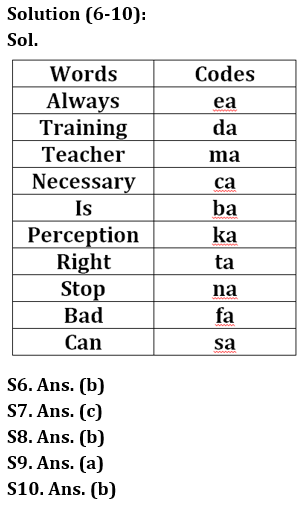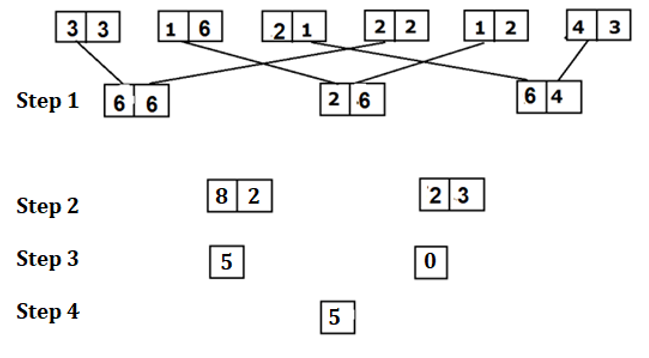# RBI Grade B Phase 1 Reasoning Quiz 2023 – 14 March

Topics- Coding- Decoding, Input-Output

Directions (1-5): Study the following information carefully and answer the questions accordingly.
The following words are coded as:
‘Reasoning Mathematics English’ is coded as ‘I#7 N#19 V#8’,
‘Word Letters Number’ is coded as ‘D@4 O#19 M@18’,
‘Actors Singers Dancers’ is coded as ‘Z@19 H#19 W@18’,
‘Obstacles Heard Riddle’ is coded as ‘L#19 S@4 I@5’,

Q1. What is the code for ‘satellite’?
(a) H#5
(b) H@5
(c) E#5
(d) E @ 5
(e) None of these

Q2. What word is coded for ‘X@25’?
(a) Crisis
(b) crying
(c) Criteria
(d) Chronology
(e) None of these

Q3. What is the code for ‘Quality Analyst’?
(a) J@25 Z#20
(b) J#25 Z#20
(c) J#25 Z@20
(d) J@25 Z@20
(e) None of these

Q4. What word is coded for ‘G#25’?
(a) Transistor
(b) Transactions
(c) Terminology
(d) Transportation
(e) None of these

Q5. What is the code for ‘verbal ability’?
(a) E#12 Z@25
(b) E@12 Z#25
(c) E@25 Z#12
(d) E#25 Z#12
(e) None of these

Directions (6-10): Study the following information carefully to answer the questions given below.
In a certain sign language,
‘Training is always necessary’ says ‘Ba Ka Da Ia’,
‘Teacher’s perception is always right’ is written ‘e ka ma ta’,
‘Teachers can stop training’ reads ‘Da Ma Na Sa’
‘Stop Bad Perception Needed’ is written as ‘Ka Fa Ka Na’.

Q6. What is the code for ‘correct’?
(a)
(b) ta
(c) What
(d) Me
(e) None of these

Q7. For which of the following words is the code ‘fa’ used?
(a) Stop
(b) Retention
(d) Required
(e) None of these

Q8. Which of the following are codes for ‘Ta Ba Fa’?
(a) Have proper training
(d) either (a) or (b)
(e) None of these

Q9. ‘A teacher is always a linguist’ might be written as ___________?
(a) Ba Ma Pa etc
(b) da ca ea ma
(c) ea ba m na
(d) Ba na ka ca
(e) None of these

Q10. What word does ‘no’ represent?
(a) Necessary
(b) Stop
(c) Correct
(d) Retention
(e) None of these

Directions (11-15): Input-output is given in different steps. In each step some mathematical operations are performed. No mathematical operations are repeated in the next step.

According to the rules followed in the above steps, find the correct step for the given input in each of the following questions.

Q11. Which of the following combinations will occur in phase III of a given input?
(a) 3, -2
(b)-2, 4
(c) 2, -1
(d) 5, 0
(E) 2, 8

Q12. Find the sum of the numbers obtained in step II?
(a) 45
(b) 48
(c) 105
(d) 57
(E) 65

Q13. Find the difference between the two numbers obtained in step III?

(a) 3
(b) 4
(c)-3
(d) 2
(E) 5

Q14. Multiplying the last step number by 4 will give the final answer?
(a) 15
(b) 20
(c) -16
(d) 12
(e) None of the above

Q15. If all the points in each block are added in step I, what will be the answer?
(a) 26
(b) 30
(c) 28
(d) 32
(e) None of the above

Solution:

Solutions (1-5):
Sol. For letters:
Reverse the first letter of each word according to the English alphabet. The antecedent of A is Z.
For symbols: @ for even letters in the word and # for odd letters in the word.
For numbers: The position value of the last letter of each word according to the English alphabet. The x-place value of A is 1.

S1. A. (a)Solutions (11-15):
1st step

1. The first digit of the first block should be multiplied with the second digit of the fourth block.
2. The second digit of the first block should be multiplied with the first digit of the fourth block.
Step 2:
1. First digit of all numbers should be added in step I and then multiply by 2 and write the value in reverse order.
2. Add the second digit of all the numbers in step I and then multiply by 2 and write the value in reverse order.
Step 3:
1. The second digit of the second block must be subtracted from the first digit of the first block.
2. The second digit of the first block must be subtracted from the second digit of the first block.
Step 4: Sum of both numbers.S11. Ans.(d)
S12. Ans.(c)
S13. Ans.(E)
S14. Ans.(b)
S15. Ans.(b)